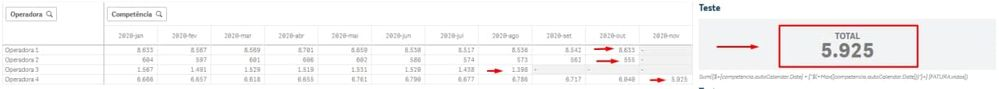# App Development

Discussion board where members can learn more about Qlik Sense App Development and Usage.

Announcements
Welcome to Qlik Community! Check out our new navigation! FIND OUT MORE
cancel
Showing results for
Did you mean:Contributor III

## Sum only the values for the last month of each customer.

Hi! Can someone help me with a tip / example on how to solve this issue:

I need to add in a KPI only the value of the last month found for each operator / customer and the months with data will not always coincide depending on the filter that the user made.

In this example: A customer has data in November, others in September, October and another in August. The formula I'm using takes only the last month of an operator / customer (in the case of the example November, which is the last month with data).Labels (2)

• ### SetAnalyisis

1 Solution

Accepted SolutionsMaster

if the requirement is for each operator, only the ones with the arrows are to be shown, you can use the following expression assuming Operator is your dimension, Date is the yearMonth and SUM(..) is your expression:

= Sum(
Aggr(
If([Date] = Max(TOTAL <[Operator]> Date), SUM([YOUR FIELD HERE]))
, [Date], Operator
)
)

this will return data for each operator where DATE = MAX(DATE) for that operator

3 RepliesMaster

if the requirement is for each operator, only the ones with the arrows are to be shown, you can use the following expression assuming Operator is your dimension, Date is the yearMonth and SUM(..) is your expression:

= Sum(
Aggr(
If([Date] = Max(TOTAL <[Operator]> Date), SUM([YOUR FIELD HERE]))
, [Date], Operator
)
)

this will return data for each operator where DATE = MAX(DATE) for that operatorContributor III
Author

Thank you! He solved the situation perfectly.Master

npTags
Community Browser